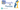# NumPy and Pandas

May 1, 2020

The NumPy library is the core library for scientific computing in Python. It provides a high-performance multidimensional array object, and tools for working with arrays. Whereas, Pandas library is built on NumPy and provides easy-to-use data structures and data analysis tools for the Python programming language.

## Installing

Numpy

• pip install numpy

Pandas

• pip install pandas

`NumPy:`

`.css-1baulvz{display:inline-block;}- creating array    - a = np.array([1,2,3])- Create an array of zeros    - np.zeros((3,4))- Create an array with random values    - np.random.random((2,2))- Create an empty array    - np.empty((3,2))- Transposing Array   - i = np.transpose(b- Append items to an array   - np.append(h,g)- Delete items from an array   - np.delete(a,)`

`Pandas:`

`- Series  - s = pd.Series([3, -5, 7, 4], index=['a', 'b', 'c', 'd'])- DataFrame   - data = {'Country': ['Belgium', 'India', 'Brazil'], 'Capital': ['Brussels', 'New Delhi', 'Brasília'],'Population': [11190846, 1303171035, 207847528]}   - df = pd.DataFrame(data,columns=['Country', 'Capital', 'Population'])- Read and Write to CSV   - pd.read_csv('file.csv', header=None, nrows=5)   - df.to_csv('myDataFrame.csv')- Read and Write to Excel   - pd.read_excel('file.xlsx')   - pd.to_excel('dir/myDataFrame.xlsx', sheet_name='Sheet1')- Info on DataFrame  - df.info()`### Rupesh Gelal

NLP and Machine Learning, Web Dev